# How to Paste Cells with Only Values \Results Keep and Remove the Formula in Excel?

We often use formulas to do calculate like sum, average for some data saved in worksheet. And most time we want to use the returned results directly for example copy it to another cell or worksheet. But we find that if we do a copy for a sum\average to another cell, we often get an error returned because of the formula is still used and for this operation we actually copy a formula instead of a value. So we need a simple way to copy\paste cell with formula included as a value. See steps below.

First prepare two lists of data, and we do sum for them. See example below: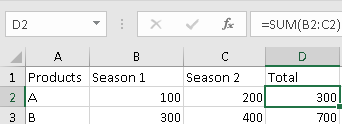Here the total value for D2 and D3 are calculated with formula, D2=SUM(B2:C2). Now we want to copy the total to another table directly. Noticed that we get 0 value. That’s because we copy the formula to H2 instead of the true value.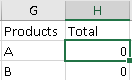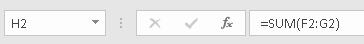We just want copy and paste the value with only result keeps but remove the formula, how can we do?

## Remove Formula and Keep Result by Paste Values Function

1. Select on D2 and D3, click Ctrl+C to do a copy.

2. Select on H2 and H3, right click your mouse, below menu is loaded, and click on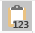icon.3. Now the results are copied to H2 and H3 with only value properly.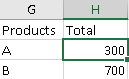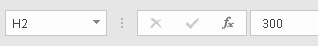Note:

You can Paste Value via Paste button in Home tab.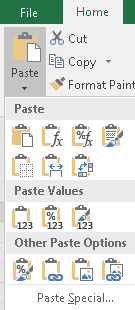You can also click on Paste Special to get more choice.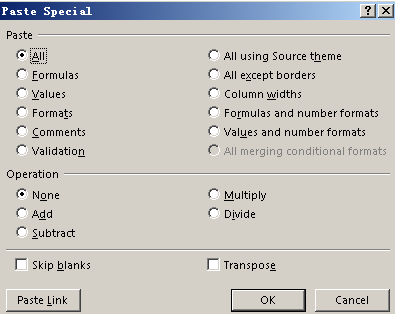Sidebar